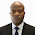## Wednesday, October 11, 2017

Several codes of practice in the world allow us to idealise structures into 2-dimensional frames for the purpose of simplified analysis. For sub-frames, it is obvious that the force method becomes less handy due to high number of redundants, and the next best alternative is the displacement method, where we solve for the unknown displacements.

In this post, we have a typical example where a problem that would have generated (21 x 21) matrix using force method has been solved using (4 x 4) matrix by displacement method. Another approach to the solution of this problem is the moment distribution method. But in this case, the displacement remains the fastest.

For the frame loaded as shown above, we have to start by drawing the kinematic basic system of the structure. This has been achieved by fixing all the nodes against rotation as shown below.

We will now have to evaluate the basic system for different cases of rotation.

Analysis of Case 1
Z1 = 1.0; Z2 = ZZ= 0

K11 = (4EI/3) + (4EI/4) + (13.6EI/3.825) = 5.889EI
K21 =  (6.8EI/3.825) = 1.778EI
K31 = 0
K41 = 0

Analysis of Case 2
Z2 = 1.0; Z2 = ZZ= 0

K12 = (6.8EI/3.825) = 1.778EI
K22 = (4EI/3) + (4EI/4) + (13.6EI/3.825) + (13.6EI/2.8) = 10.7460EI
K32 = (6.8EI/2.8) =  2.4286EI
K42 = 0

Analysis of Case 3
Z3 = 1.0; Z1 = ZZ= 0

K13 = 0
K23 = (4EI/3) + (4EI/4) + (13.6EI/3.825) + (13.6EI/2.8) = 10.7460EI= 2.4286EI
K33 = (4EI/3) + (4EI/4) + (13.6EI/3.325) + (13.6EI/2.8) = 11.2807EI
K43 = (6.8EI/3.325) = 2.0451EI

Analysis of Case 4
Z4 = 1.0; Z1 = ZZ= 0

K14 = 0
K24 = 0
K34 =  (6.8EI/3.325) = 2.045EI
K44 = (4EI/3) + (4EI/4) + (13.6EI/3.325) = 6.4236EI

Stiffness coefficient due to externally applied load;

K1P = -(q1L12/12) = -(26.148 × 3.8252) / 12 = -31.880 KNm

K2P = (q1L12/12) - (q2L22/12) = [(26.148 × 3.8252) / 12] - [(25.437 × 2.802) / 12] = 15.261 KNm

K3P = (q2L22/12) - (q3L32/12) = [(25.437 × 2.82) / 12] - [(27.345 × 3.3252) / 12] = -8.5741 KNm

K1P = (q3L32/12) = (27.345 × 3.3252) / 12 = 25.193 KNm

The appropriate cannonical equation;

K11Z1 + K12Z2 + K13Z3 + K14Z4 + K1P = 0
K21Z1 + K22Z2 + K23Z3 + K24Z4 + K2P = 0
K31Z1 + K32Z2 + K33Z3 + K34Z4 + K3P = 0
K41Z1 + K42Z2 + K43Z3 + K44Z4 + K4P = 0

On substituting;

5.889Z1 + 1.778Z2     +    0Z3        + 0Z4         = 31.880
1.778Z1 + 10.746Z2   + 2.4286Z3  + 0Z4         = -15.261
0Z1        + 2.4286Z2   + 11.287Z3  + 2.045Z4  = 8.5741
0Z1        + 0Z2            + 2.045Z3  + 6.4236Z4  = -25.193

On solving;
Z1 = 6.3102/EI (radians)
Z2 = -2.9701/EI (radians)
Z3 = 2.2384/EI (radians)
Z4 = -4.6346/EI (radians)

Now that we have obtained the rotations, we can now substitute and obtain the moments at the critical points;

Mi = M1Z1 + M2Z2 + M3Z3 + M4Z4 + P

Bottom column support moments
MA = (6.3102/EI) × (2EI/4) = 3.1551 KNm
MB = (-2.9701/EI) × (2EI/4) = -1.485 KNm
MC = (2.2384/EI) × (2EI/4) = 1.1192 KNm
MD = (-4.6346/EI) × (2EI/4) = -2.3173 KNm

Beam Support Moments
M1R = [(6.3102/EI) × (13.6EI/3.825)] - [(2.9701/EI) × (6.8EI/3.825)] - 31.880 = -14.7239 KNm

M2L = [(6.3102/EI) × (6.8EI/3.825)] - [(2.9701/EI) × (13.6EI/3.825)] + 31.880 = 32.537 KNm

M2R = [-(2.9701/EI) × (13.6EI/2.8)] + [(2.23841/EI) × (6.8EI/2.8)] - 16.619 = -25.6090 KNm

M3L = [-(2.9701/EI) × (6.8EI/2.8)] + [(2.2384/EI) × (13.6EI/2.8)] + 16.619 = 20.278 KNm

M4R = [(2.2384/EI) × (13.6EI/3.325)] - [(4.6346/EI) × (6.8EI/3.325)] - 25.193 = -25.5157 KNm

M3L = [(2.2384/EI) × (6.8EI/3.325)] - [(4.6346/EI) × (13.6EI/3.325)] + 25.193 = 10.814 KNm

Thank you for visiting Structville today....
Our Facebook page is at www.facebook.com/structville

#### 6 comments:

1.Hello Bro,

The coefficient stiffness 13.6 i'm not seeing where it's derived from? Can you explain how you derived it? thanks

Fc

1.This comment has been removed by the author.

2.Sorry that I did not make the post very 'down to earth'. The moment from unit rotation at any near end is given by 4EI/L and at any far end is 2EI/L.

So 4 x 3.4EI = 13.6EI/L

3.The beam stiffness was given, I missed that! Just to be clear, the beam stiffness is factored up due to the column stiffness, this is my understand.

What if the upper column had a smaller stiffness compared to the lower column would it be and average factor times the beam stiffness?

Thanks
Frank

4.No, there is nothing like average in handling relative stiffness of Structural members. Note that there is no rule that you must work in terms of column stiffness. You can also work in terms of beam stiffness.

For example, if the upper column has lesser stiffness, we can have something like; 0.75EI for upper column, EI for lower column, and 3.4 EI for beams.

5.